Zen Pharaohs of Ultimate Love mathematics, music, science, wood....what else could you ask?Next: Meixner Functions and TIB Up: Recursive Windowing of Time Previous: Comparison With a Rectangular

# Triangular Input Balanced Realizations

In a realization of the state space form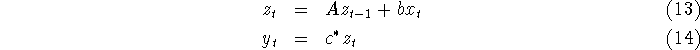the impulse response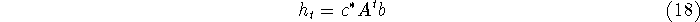is preserved by the action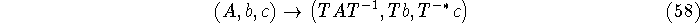that is,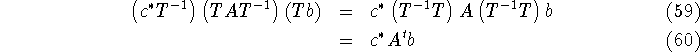where T is a nonsingular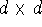matrix. This corresponds to the change of coordinates of the state vector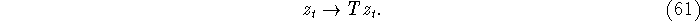When we choose the filter parameters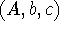we can exploit this symmetry to obtain choices which, although having the same signal properties, have superior numerical or computational properties. Since these realizations have the same impulse response, they are called observationally equivalent.

After imposing some more or less unobjectionable constraints on the filter, it turns out that any two observationally equivalent systems are related by this type of coordinate change. Note: we should go into minimality, observability and reachability in somewhat more detail here.

A system of dimension d is called minimal if it is not possible to realize the impulse response with a system of lower dimension. This means that each component of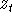actually is used at some point, and that the distribution ofvectors actually fills up d-dimensions. However, it can happen that a minimal realization of an impulse response involves coordinates forin which the vectorsare distributed in such a way that the variation in some directions is very small compared to others, i.e. that the ellipsoids of constant probability are very far from spheres. It should not be too surprising that this can expose a finite precision realization of the system to numerical pathologies. It is therefore desirable that all the components ofhave similar scaling.

It is impossible to obtain this property without any information about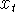. However, if it is known thatis relatively noisy, then it is possible to get good results by choosing the coordinates forin such a way thatwould be spherically symmetrically distributed if thewere independent and identically distributed. It turns out that this means choosing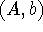so that Stein's equation is satisfied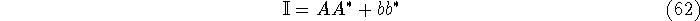and determining c from the system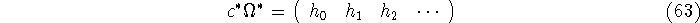where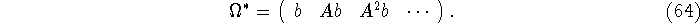Note that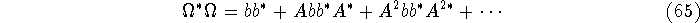but that thanks to the Stein equation, we have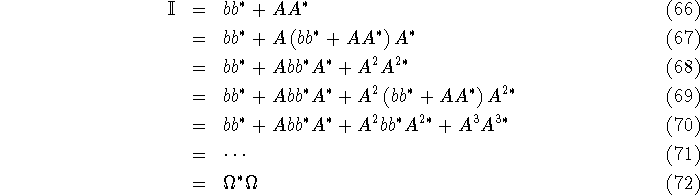which converges as long as A is stable. (We are only interested in stable A, so we can always use this). Since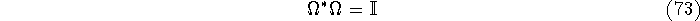it follows that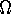is column unitary, and we can solve for the least squares approximation to c byThis corresponds to expanding the impulse response in the orthogonal functions given by the columns of, and the `Fourier' coefficients of the impulse response are the components of c.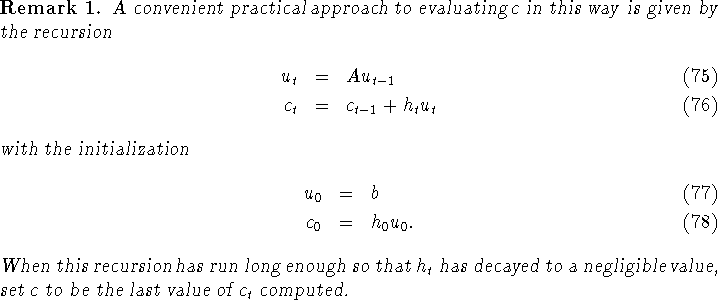We have not exhausted the possible choices of coordinates for, since by Shur's decomposition, we can always arrange for A to be triangular with a unitary choice of T. The unitarity of T preserves the Stein equation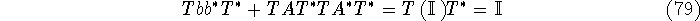and we use this choice to reduce A to a triangular matrix. A system wheresatisfy the Stein equation with A triangular is called a Triangular Input Balanced (TIB) system. This choice is particularly good since in addition to the good numerical properties implied by the Stein equation, it is possible to compute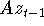in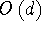operations, as opposed to the usual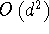for general A.Next: Meixner Functions and TIB Up: Recursive Windowing of Time Previous: Comparison With a Rectangular

Fri Jun 27 03:10:38 EDT 1997